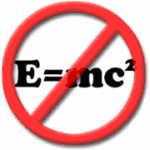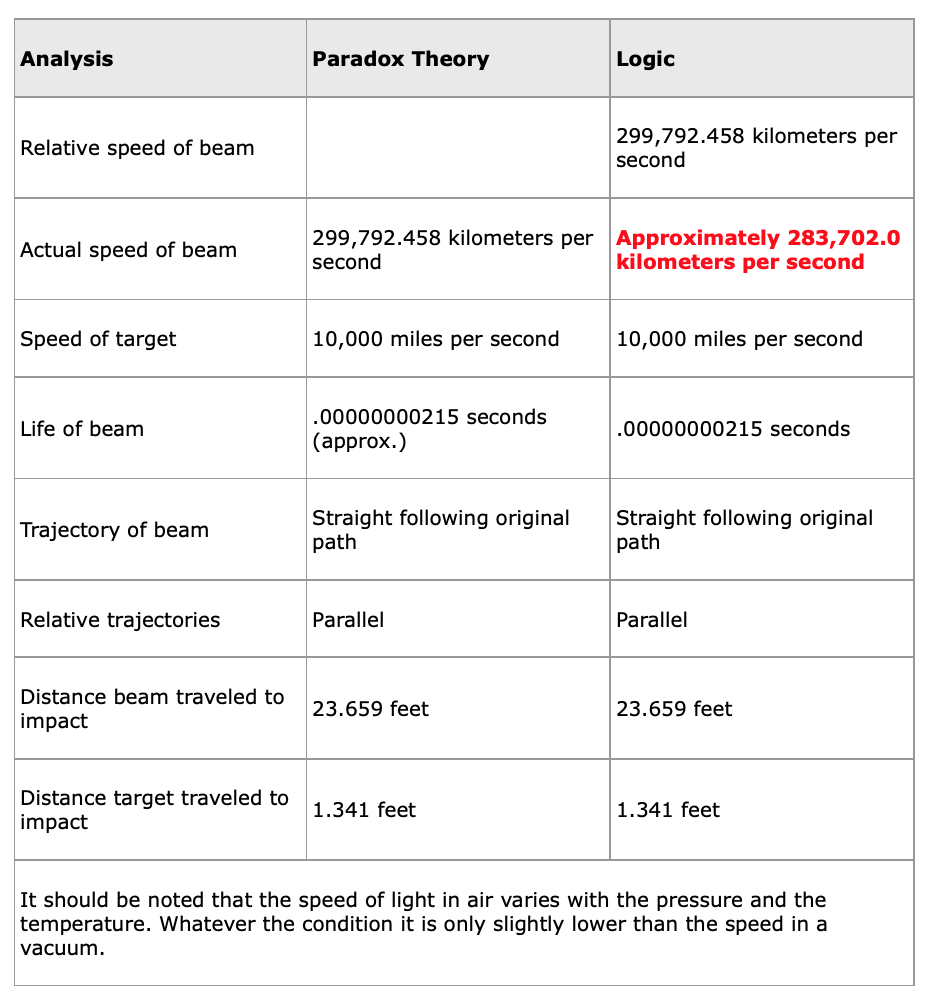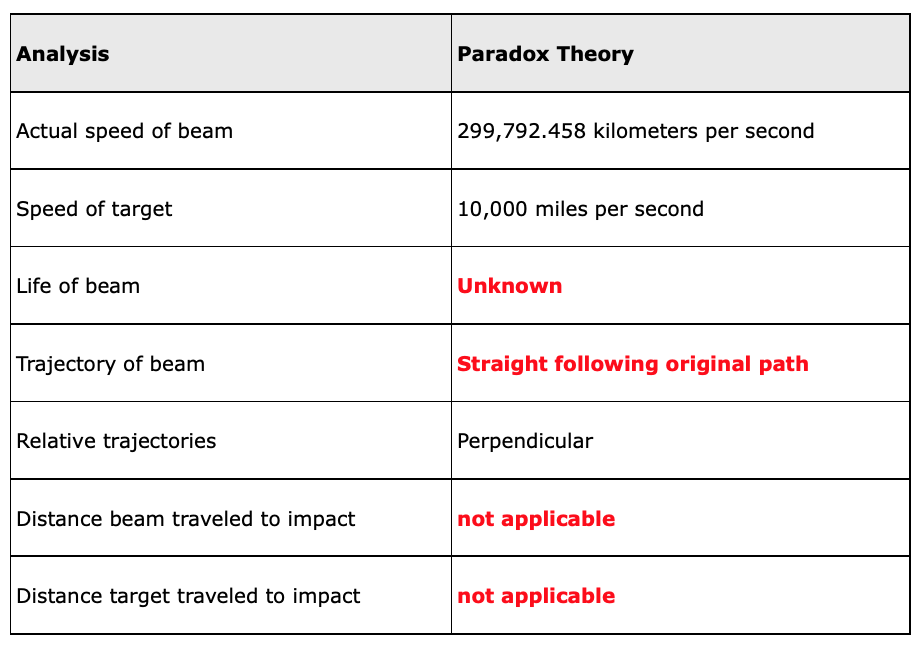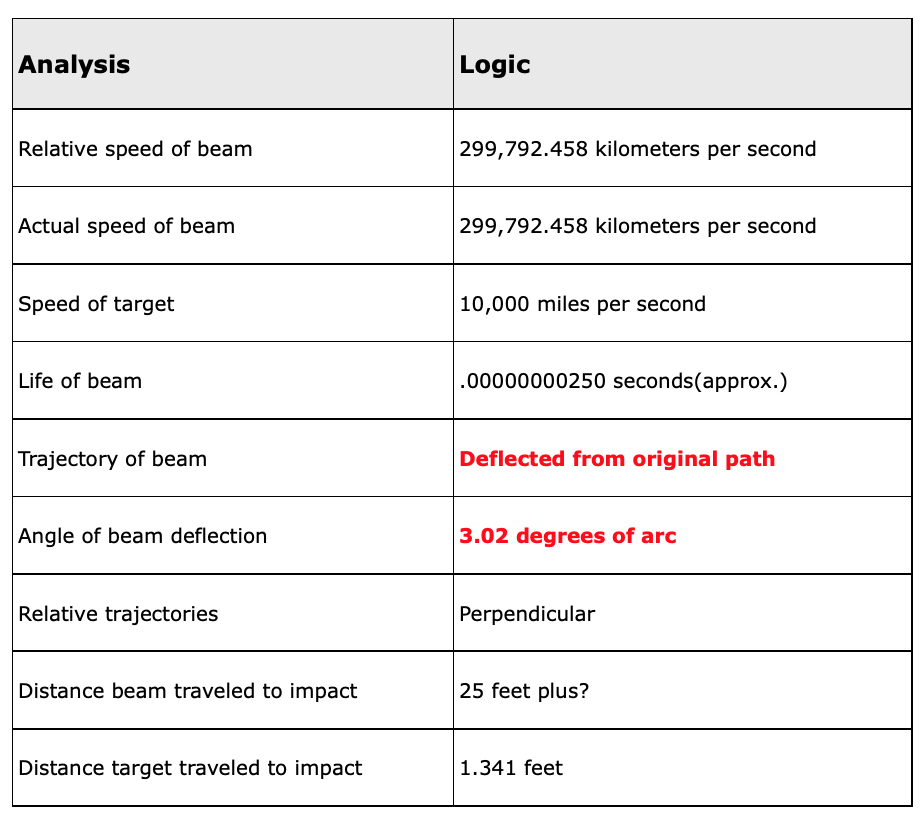Select PageThe Laser Drag Experiments.
by Murray Tovi

These experiments prove that the Big Bang and the paradox speed of light theories are incompatible and cannot both be true. The experiments specifically prove that if the Milky Way is moving at a minimum of 1000 kilometers per second away from the Big Bang then there can be no paradox in the movement of light and further that it must be natural and relative. If you are unfamiliar with these theories click on the research links at bottom.

We begin by assuming, based on red-shift analysis, that the Big Bang occurred over ten billion years ago and that all galaxies are fleeing that event at incredible speeds. Future experiments will use a much lower minimum Milky Way velocity than 1000 kilometers per second such as the estimated Sun’s velocity of 217 kilometers per second to corroborate the conclusions found at 1000 kilometers per second.

To begin, simple Algebra teaches that if you are in a car moving at 60 mph and another car passes you in the opposite direction at a relative speed of 120 mph you can deduce that the other car is moving at 60 mph in actual speed.

Yet when we measure the speed of light from anywhere on Earth, knowing Earth is in complicated motion we conclude that there is no such thing as relative speed when measuring the speed of light. The phenomenon remains at one measured speed in a vacuum (as does the entire electromagnetic spectrum) irrespective of the velocity or relative direction of the observer (the measurement taker).

Albert Einstein utilizes this phenomenon in his speed of light paradox theory which claims that light (in a vacuum) moves away from any moving object at 299,792.458 kilometers per second faster than the object is moving while only moving at 299,792.458 kilometers per second.

In opposition, these two experiments prove that if the Milky Way is moving at a minimum of 1000 kilometers per second, then the paradox theory must be invalid and that the observed speed of light is an illusion caused by a natural phenomenon distorting our perspective.

The laser drag experiment was conducted in an open field in central Florida and consisted of a construction laser used for leveling and a foot square white target separated by 25 feet. Both were secured to the ground by posts set in concrete making them immobile. The beam when turned on would move at a 45 degree angle to the longitudinal and latitudinal lines of Earth. The graphic below alters the proportions of the distances and objects for illustration purposes only. It has no effect on the outcome of the experiment.

On October 17, 2000 the laser was turned on and kept on for 72 hours allowing the Earth three full revolutions at 1000 miles per hour (at the Equator), three days at 18 miles per second orbital trajectory around the Sun and three days of the Sun’s orbital trajectory at 217 kilometers per second miles per second around the center of the galaxy, three days of the galaxy’s velocity as part of a local group of galaxies moving toward the Constellation Hydra somewhere between 300 and 600 kilometers per second and three days of the Galaxy’s escape velocity from the Big Bang at some unknown, but likely, tens of thousands of kilometers per second and in some unknown direction.

It was assumed that with all this complicated movement, at some point in the 72 hours, the laser beam would be near parallel to the line of the Milky Way’s assumed escape trajectory from the Big Bang and at another time near perpendicular to that line.

Since we cannot red-shift ourselves in order to get some sense of the Milky Way’s velocity and since we needed a number to evaluate the experiment 10,000 miles per second was chosen.

The farthest and fastest receding galaxy is moving at 61,000 kilometers per second away from us or is it us from them or is it each from each other at 30,500 kilometers per second, etc. As such the gauge could have just as easily been set anywhere from 1000 kilometers per second to just below the speed of light without altering the experiment’s principle conclusions.

Since the laser beam stayed on the center of the target throughout the 72 hours we can conclude that the beam stayed on center when the beam was perpendicular or parallel to the expansion line (the trajectory of the Milky Way), points assumed reached somewhere in the 33 night journey.

On that set of assumptions the experiment is analyzed.

It should be noted that the target center in the following animations is defined as a circle having a 3/8th inch radius from the center of the beam’s initial impact on the target. This is slightly larger than the diameter of the beam in order to adjust for temperature variations, weather conditions, battery changes, etc.

Animation 1 follows a section of the laser beam from the time it leaves the laser gun to the time it hits the center of the target while the laser beam is near parallel with the Milky Way’s escape trajectory assumed reached at some point in the 33 night journey. It is analyzed from both the paradox theory and by applying logic following the same laws governing relative measurements of bodies in motion as indicated in the moving car examples above.

Animation 2 applies the paradox theory when the beam is near perpendicular to the expansion line assumed reached at some point in the 33 night journey.

Animation 3 applies logic when the beam is near perpendicular to the expansion line assumed reached at some point in the 33 night journey.

It should be noted that the relative speed of the laser beam and the speed of the target is altered for illustration purposes. This has no bearing on the outcome.

Animation 1, 2, 3, 4 Below with AnalysisIn the second analysis the beam missed the target center by over one foot using a 10,000 miles per second gauge. Using 1000 kilometers per second as the gauge the target center in the second analysis would miss the target center by approximately a full inch, well outside its boundary.

Albert Einstein predicted that starlight passing near the surface of the Sun would be deflected by its colossal gravity. He estimated the deflection at 1/3600th of a degree of arc. This was confirmed in the 1919 and 1922 solar eclipse observations.

However, the measured deflection in the laser drag experiment appears to be exponentially greater. Though the beam is moving laterally in the last animation as it is moving forward, it appears straight to our tandem moving perspective. Further, it would appear that the beam at any other angle throughout the 72 hours would be both deflected and either pushed up or pushed down in velocity in varying predictable and exacting combinations.

To summarize:

If the Universe is expanding then light behaves naturally, not paradoxically. Measurements of the speed of light and observations of its trajectory are, in almost all instances, illusions caused by a natural phenomenon. Like gravity, this phenomenon is invisible and everywhere in the sky. It is moving, like Earth’s gravitational field, somewhere between 1000 kilometers per second and just below the speed of light.

On June 27, 2002 the laser drag experiment was repeated with modifications to discover any flaws in the first experiment. The experiment lasted four days as now four targets were positioned in a circumference 33 feet away from a single laser. The laser was rotated every twenty four hours to move from the center of one target to the center of the next one. Two targets were positioned at 45 degrees angles to the longitudinal and latitudinal lines of the Earth so each beam would follow a path of 90 degrees to the other. The third target was positioned parallel to the longitudinal line and the final one parallel to the latitudinal line. Again the target center(s) were continuously impacted through each 24 hour cycle.

These results corroborate the results of the October 20-22, 2000 experiment. As mentioned earlier the next experiment will lower the 1000 kilometer per second minimum to 217 kilometers per second. The target will, in this new experiment be 100 feet away from the laser gun.

This concludes The Laser Drag Experiments.

Tovi Sciences
A fatal flaw in the laws of physics
(A scientific study redefining the structure of the Universe)